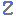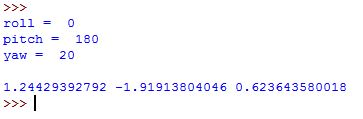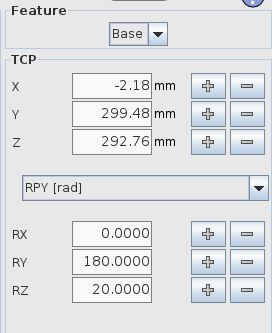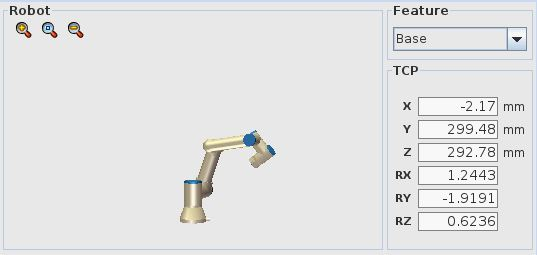Visit Zacobria Webshop

Python code example of converting RPY/Euler angles to Rotation Vector/Angle Axis for Universal-Robots.

Python code example of converting RPY/Euler angles to Rotation Vector/Angle Axis for Universal-Robots.

Mind you that when all input values are 0, there will be a division by zero error at this line:
# multi = 1 / (2 * math.sin(theta))
In this case theta will be 0 and the sin of 0 is 0, so you’ll probably have to make a special case for this and set the rx, ry and rz to 0 directly.

```import math
import numpy as np

roll = 2.6335
pitch = 0.4506
yaw = 1.1684

print "roll = ", roll
print "pitch = ", pitch
print "yaw = ", yaw
print ""

yawMatrix = np.matrix([
[math.cos(yaw), -math.sin(yaw), 0],
[math.sin(yaw), math.cos(yaw), 0],
[0, 0, 1]
])

pitchMatrix = np.matrix([
[math.cos(pitch), 0, math.sin(pitch)],
[0, 1, 0],
[-math.sin(pitch), 0, math.cos(pitch)]
])

rollMatrix = np.matrix([
[1, 0, 0],
[0, math.cos(roll), -math.sin(roll)],
[0, math.sin(roll), math.cos(roll)]
])

R = yawMatrix * pitchMatrix * rollMatrix

theta = math.acos(((R[0, 0] + R[1, 1] + R[2, 2]) - 1) / 2)
multi = 1 / (2 * math.sin(theta))

rx = multi * (R[2, 1] - R[1, 2]) * theta
ry = multi * (R[0, 2] - R[2, 0]) * theta
rz = multi * (R[1, 0] - R[0, 1]) * theta

print rx, ry, rz

```

Testing angle calculation and compare to robot values.

RPY values tested

roll = 0
pitch = 180
yaw = 20

```import math
import numpy as np

#roll = 2.6335
#pitch = 0.4506
#yaw = 1.1684

roll = 0
pitch = 180
yaw = 20

print "roll = ", roll
print "pitch = ", pitch
print "yaw = ", yaw
print ""

yawMatrix = np.matrix([
[math.cos(yaw), -math.sin(yaw), 0],
[math.sin(yaw), math.cos(yaw), 0],
[0, 0, 1]
])

pitchMatrix = np.matrix([
[math.cos(pitch), 0, math.sin(pitch)],
[0, 1, 0],
[-math.sin(pitch), 0, math.cos(pitch)]
])

rollMatrix = np.matrix([
[1, 0, 0],
[0, math.cos(roll), -math.sin(roll)],
[0, math.sin(roll), math.cos(roll)]
])

R = yawMatrix * pitchMatrix * rollMatrix

theta = math.acos(((R[0, 0] + R[1, 1] + R[2, 2]) - 1) / 2)
multi = 1 / (2 * math.sin(theta))

rx = multi * (R[2, 1] - R[1, 2]) * theta
ry = multi * (R[0, 2] - R[2, 0]) * theta
rz = multi * (R[1, 0] - R[0, 1]) * theta

print rx, ry, rz```Rx – Ry and Rz show the same values as calculated by the Python program.Disclaimer: While the Zacobria Pte. Ltd. believes that information and guidance provided is correct, parties must rely upon their skill and judgement when making use of them. Zacobria Pte. Ltd. assumes no liability for loss or damage caused by error or omission, whether such an error or omission is the result of negligence or any other cause. Where reference is made to legislation it is not to be considered as legal advice. Any and all such liability is disclaimed.

If you need specific advice (for example, medical, legal, financial or risk management), please seek a professional who is licensed or knowledgeable in that area.

Author:
By Zacobria Lars Skovsgaard
Accredited 2015-2018 Universal Robots support Centre and Forum.